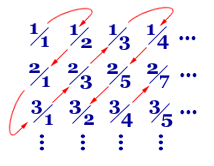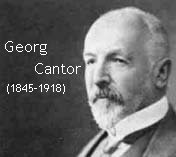## Saturday, April 11, 2009

### To Infinity and Beyond!Are there different sizes of Infinity???? Surprisingly, YES!

It comes down to whether or not we can “count” the infinite set so that we can go down a “checklist” that contains each number, and progress down that list so that we come to (eventually) every number in the set in a sequential order. For some infinite number sets, this is possible, but for other infinite number sets, it is not possible. An infinite number set is not countable, then it is infinitely larger than an infinite number set that IS countable!!! Sound paradoxical? Here’s some examples:

The set of Natural Numbers {1, 2, 3, . . .} is infinite, yet “countable.” That is, the numbers continue in that pattern forever, but we can start at 1 and check off numbers down the list. The Whole Numbers are the same, except they start with zero, so they have one extra element in their set. Despite this, mathematicians say that they are actually the same “size,” or have the same cardinality. In general, a mathematician would say that set A has "just as many" objects as set B if the objects in A and B can be put into one-to-one correspondence with each other.

What about the Integers??? Recall these are the positive and negative Natural Numbers, including zero, which means they progress infinitely in BOTH the positive and negative direction. Can we list them in a checklist format? With a little ingenuity, we can! Starting with zero, we get: {0,-1, 1, -2, 2, -3, 3, . . . }. We simply list them like the Whole numbers except with consecutive -/+ pairs. So the Integers are Infinite, yet countable, and so, believe it or not, are mathematically the same “size” as the Natural Numbers, and NOT, as you might think, at least twice as big!!!!

It gets trickier when we look at the Rational Numbers, thenumbers comprised of the quotient of two Integers (also known as the fractions). Now every Integer is a Rational (just put that Integer over the number 1) so we say that the Integers are a subset of the Rationals, just like the Whole Numbers are a subset of the Integers. Arranging the densely populated Rationals in a one-directional, countable way is not as intuitive, yet still possible. The trick is to arrange them in a double, square array, then zigzagging along the diagonals of the square, as in the diagram at right.

Isn’t it fun to be clever?

The beauty and art of mathematics is that it helps us, through precise, rigorous definitions, reconcile issues when our intuition fails, and with infinity, it usually does. A mathematician named Georg Cantor tried to make precise the notion of the size of an infinite set.
The essence of mathematics lies in its freedom.”—Georg CantorNext time: he set of Real Numbers--Countable or Uncountable???

An original Limerick for the occasion:
Of the infinite countable size,It makes sense, although wrong, to surmise,
That if one set has more
It is bigger, therefore.
“For they’re equal!” said Cantor, “SURPRISE!!”

#### 6 comments:bob s said...

Sometimes (but not real often) I wonder what it might have been like to know one of our famous mathematicians. Would they have been infintely engaging or infinitely boring?

Andrew said...

I remember our lesson on infinity, I think it was in chapter P. That was an awesome lesson, I thought it was clever how infinity works and even cleverer how mathematicians can count it. Good post, Korpi!

kwkorpi said...

bob, your comment reminds me of a joke at a mathematician's expense:

Q: What is the difference between an introverted and an extroverted mathematician??

A: An extroverted mathematician will look at your shoes when he talks to you.

Freckles said...

I actually JUST heard that joke this week... at work... only the word "actuary" was substituted for "mathematician" :)

Kirby said...

Infinity is a cool concept, though sometimes I find it hard to comprehend. It's not classified as a 'number' per se, but an idea. *shakes head* The symbol for infinity, an eight on it's side, is also slightly misleading, in my opinion. If one were to number it like a number line, it would eventually loop back to the beginning... In real life, infinity is better represented as a straight line that continues forever in each direction...LC said...

Bob, I think we do know a famous mathematician.....except right now he's only famous to us. I think the answer to your question is infinetly engaging.

Speaking of countable....wow on the number of posters today.